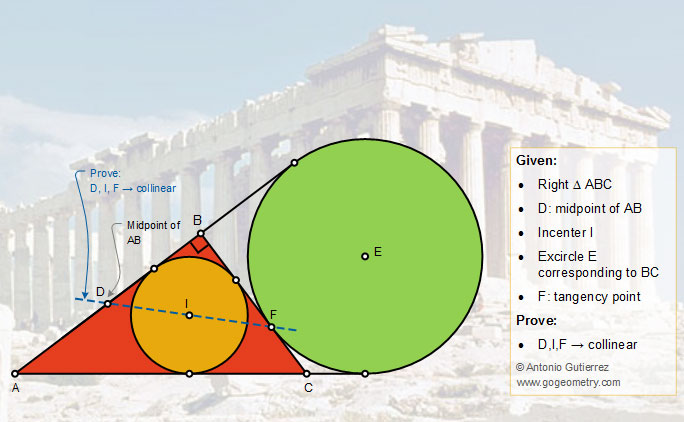# Geometry Problem 1408: Right Triangle, Incircle, Excircle, Incenter, Midpoint, Tangency Point, Collinearity

### Proposition

The figure below shows a right triangle ABC (angle B = 90 degree). The excircle E corresponding to BC is tangent to BC at F. If I is the incenter and D is the midpoint of AB, prove that D, I, and F are collinear.